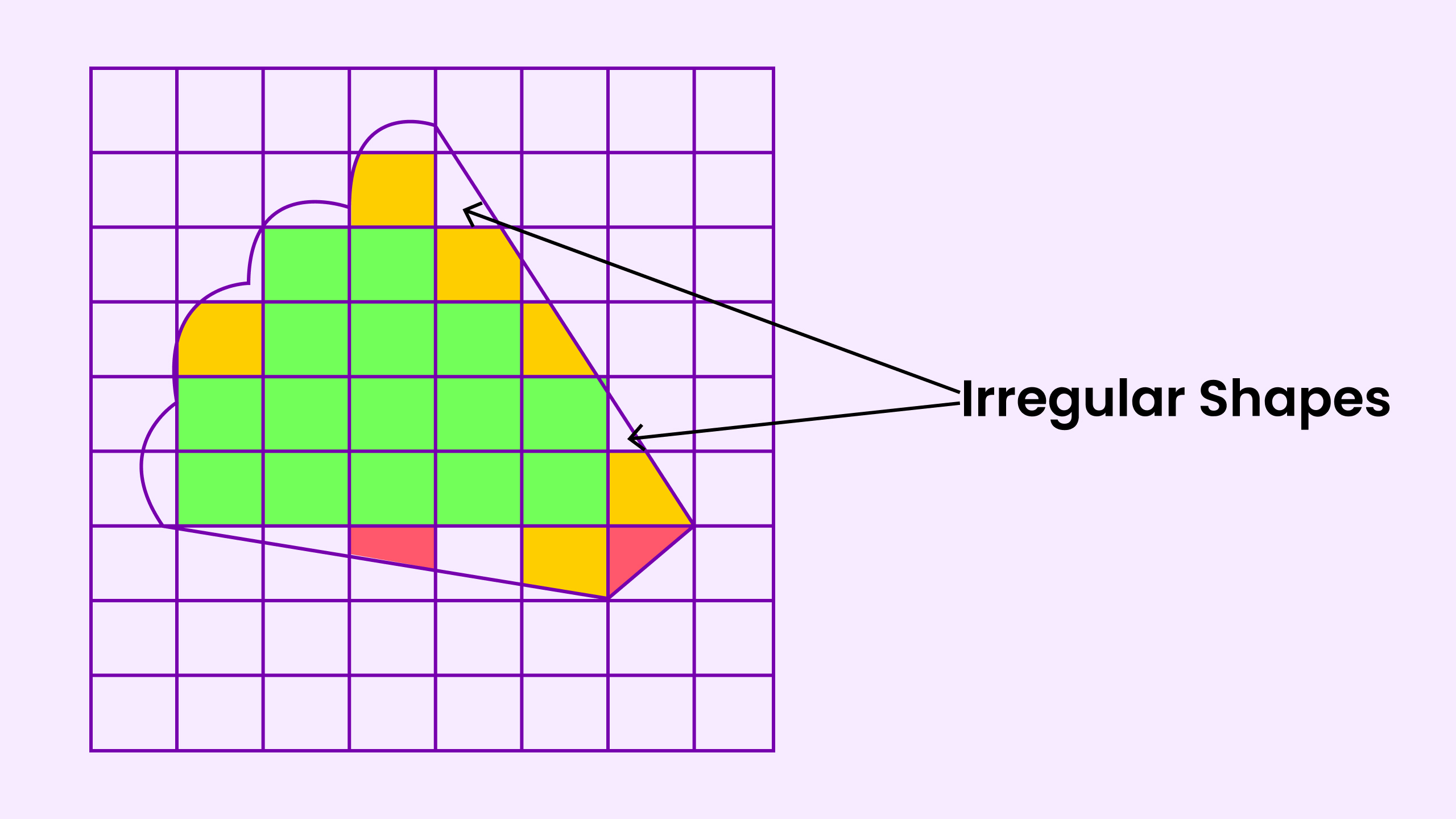category: UIUX 360

# Irregular ShapesScroll Down## What is Irregular Shapes?

### Overview

Irregular shapes are closed polygons with five or more sides of varying lengths. These figures can be further divided or broken down into triangles, squares, and quadrilaterals to evaluate the area. Any figure that shows slight symmetry on one or more axes.

Irregular shapes are closed polygons with five or more sides of varying lengths. These figures can be further broken down into triangles, squares, and quadrilaterals to evaluate the area. We can apply this technique to curved shapes other than perfect circles or semicircles and irregular quadrilaterals. In this method, divide the figure into unit squares.

The total number of unit squares within the figure determines the total area. Using this method for any irregular shapes, we can predefined formulas to calculate the area of such figures and add them together to get the total area.### Need help to develop a similar project?

Book a call with us and get the party started!